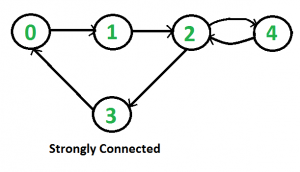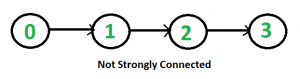Open in App
Not now

# Check if a graph is strongly connected | Set 1 (Kosaraju using DFS)

• Difficulty Level : Medium
• Last Updated : 27 Jan, 2023

Given a directed graph, find out whether the graph is strongly connected or not. A directed graph is strongly connected if there is a path between any two pair of vertices. For example, following is a strongly connected graph.It is easy for undirected graph, we can just do a BFS and DFS starting from any vertex. If BFS or DFS visits all vertices, then the given undirected graph is connected. This approach won’t work for a directed graph. For example, consider the following graph which is not strongly connected. If we start DFS (or BFS) from vertex 0, we can reach all vertices, but if we start from any other vertex, we cannot reach all vertices.How to do for directed graph?

A simple idea is to use a all pair shortest path algorithm like Floyd Warshall or find Transitive Closure of graph. Time complexity of this method would be O(v3).
We can also do DFS V times starting from every vertex. If any DFS, doesn’t visit all vertices, then graph is not strongly connected. This algorithm takes O(V*(V+E)) time which can be same as transitive closure for a dense graph.

A better idea can be Strongly Connected Components (SCC) algorithm. We can find all SCCs in O(V+E) time. If number of SCCs is one, then graph is strongly connected. The algorithm for SCC does extra work as it finds all SCCs.

Following is Kosaraju’s DFS based simple algorithm that does two DFS traversals of graph:

1. Initialize all vertices as not visited.
2. Do a DFS traversal of graph starting from any arbitrary vertex v. If DFS traversal doesn’t visit all vertices, then return false.
3. Reverse all arcs (or find transpose or reverse of graph)
4. Mark all vertices as not-visited in reversed graph.
5. Do a DFS traversal of reversed graph starting from same vertex v (Same as step 2). If DFS traversal doesn’t visit all vertices, then return false. Otherwise return true.

The idea is, if every node can be reached from a vertex v, and every node can reach v, then the graph is strongly connected. In step 2, we check if all vertices are reachable from v. In step 4, we check if all vertices can reach v (In reversed graph, if all vertices are reachable from v, then all vertices can reach v in original graph).
Following is the implementation of above algorithm.
Implementation:

## C++

 `// C++ program to check if a given directed``// graph is strongly connected or not``#include ``#include ``#include ``using` `namespace` `std;` `class` `Graph``{``    ``int` `V;    ``// No. of vertices``    ``list<``int``> *adj; ``// An array of adjacency lists` `    ``// A recursive function to print DFS``    ``// starting from v``    ``void` `DFSUtil(``int` `v, ``bool` `visited[]);``public``:``    ``// Constructor and Destructor``    ``Graph(``int` `V) { ``this``->V = V;  adj = ``new` `list<``int``>[V];}``    ``~Graph() { ``delete` `[] adj; }` `    ``// Method to add an edge``    ``void` `addEdge(``int` `v, ``int` `w);` `    ``// The main function that returns true if the``    ``// graph is strongly connected, otherwise false``    ``bool` `isSC();` `    ``// Function that returns reverse (or transpose)``    ``// of this graph``    ``Graph getTranspose();``};` `// A recursive function to print DFS starting from v``void` `Graph::DFSUtil(``int` `v, ``bool` `visited[])``{``    ``// Mark the current node as visited and print it``    ``visited[v] = ``true``;` `    ``// Recur for all the vertices adjacent to this vertex``    ``list<``int``>::iterator i;``    ``for` `(i = adj[v].begin(); i != adj[v].end(); ++i)``        ``if` `(!visited[*i])``            ``DFSUtil(*i, visited);``}` `// Function that returns reverse (or transpose) of this graph``Graph Graph::getTranspose()``{``    ``Graph g(V);``    ``for` `(``int` `v = 0; v < V; v++)``    ``{``        ``// Recur for all the vertices adjacent to this vertex``        ``list<``int``>::iterator i;``        ``for``(i = adj[v].begin(); i != adj[v].end(); ++i)``        ``{``            ``g.adj[*i].push_back(v);``        ``}``    ``}``    ``return` `g;``}` `void` `Graph::addEdge(``int` `v, ``int` `w)``{``    ``adj[v].push_back(w); ``// Add w to v’s list.``}` `// The main function that returns true if graph``// is strongly connected``bool` `Graph::isSC()``{``    ``// St1p 1: Mark all the vertices as not visited``    ``// (For first DFS)``    ``bool` `visited[V];``    ``for` `(``int` `i = 0; i < V; i++)``        ``visited[i] = ``false``;` `    ``// Step 2: Do DFS traversal starting from first vertex.``    ``DFSUtil(0, visited);` `     ``// If DFS traversal doesn’t visit all vertices,``     ``// then return false.``    ``for` `(``int` `i = 0; i < V; i++)``        ``if` `(visited[i] == ``false``)``             ``return` `false``;` `    ``// Step 3: Create a reversed graph``    ``Graph gr = getTranspose();` `    ``// Step 4: Mark all the vertices as not visited``    ``// (For second DFS)``    ``for``(``int` `i = 0; i < V; i++)``        ``visited[i] = ``false``;` `    ``// Step 5: Do DFS for reversed graph starting from``    ``// first vertex. Starting Vertex must be same starting``    ``// point of first DFS``    ``gr.DFSUtil(0, visited);` `    ``// If all vertices are not visited in second DFS, then``    ``// return false``    ``for` `(``int` `i = 0; i < V; i++)``        ``if` `(visited[i] == ``false``)``             ``return` `false``;` `    ``return` `true``;``}` `// Driver program to test above functions``int` `main()``{``    ``// Create graphs given in the above diagrams``    ``Graph g1(5);``    ``g1.addEdge(0, 1);``    ``g1.addEdge(1, 2);``    ``g1.addEdge(2, 3);``    ``g1.addEdge(3, 0);``    ``g1.addEdge(2, 4);``    ``g1.addEdge(4, 2);``    ``g1.isSC()? cout << ``"Yes\n"` `: cout << ``"No\n"``;` `    ``Graph g2(4);``    ``g2.addEdge(0, 1);``    ``g2.addEdge(1, 2);``    ``g2.addEdge(2, 3);``    ``g2.isSC()? cout << ``"Yes\n"` `: cout << ``"No\n"``;` `    ``return` `0;``}`

## Java

 `// Java program to check if a given directed graph is strongly``// connected or not``import` `java.io.*;``import` `java.util.*;``import` `java.util.LinkedList;` `// This class represents a directed graph using adjacency``// list representation``class` `Graph``{``    ``private` `int` `V;   ``// No. of vertices``    ``private` `LinkedList adj[]; ``//Adjacency List` `    ``//Constructor``    ``Graph(``int` `v)``    ``{``        ``V = v;``        ``adj = ``new` `LinkedList[v];``        ``for` `(``int` `i=``0``; i i = adj[v].iterator();``        ``while` `(i.hasNext())``        ``{``            ``n = i.next();``            ``if` `(!visited[n])``                ``DFSUtil(n,visited);``        ``}``    ``}` `    ``// Function that returns transpose of this graph``    ``Graph getTranspose()``    ``{``        ``Graph g = ``new` `Graph(V);``        ``for` `(``int` `v = ``0``; v < V; v++)``        ``{``            ``// Recur for all the vertices adjacent to this vertex``            ``Iterator i = adj[v].listIterator();``            ``while` `(i.hasNext())``                ``g.adj[i.next()].add(v);``        ``}``        ``return` `g;``    ``}` `    ``// The main function that returns true if graph is strongly``    ``// connected``    ``Boolean isSC()``    ``{``        ``// Step 1: Mark all the vertices as not visited``        ``// (For first DFS)``        ``Boolean visited[] = ``new` `Boolean[V];``        ``for` `(``int` `i = ``0``; i < V; i++)``            ``visited[i] = ``false``;` `        ``// Step 2: Do DFS traversal starting from first vertex.``        ``DFSUtil(``0``, visited);` `        ``// If DFS traversal doesn't visit all vertices, then``        ``// return false.``        ``for` `(``int` `i = ``0``; i < V; i++)``            ``if` `(visited[i] == ``false``)``                ``return` `false``;` `        ``// Step 3: Create a reversed graph``        ``Graph gr = getTranspose();` `        ``// Step 4: Mark all the vertices as not visited (For``        ``// second DFS)``        ``for` `(``int` `i = ``0``; i < V; i++)``            ``visited[i] = ``false``;` `        ``// Step 5: Do DFS for reversed graph starting from``        ``// first vertex. Starting Vertex must be same starting``        ``// point of first DFS``        ``gr.DFSUtil(``0``, visited);` `        ``// If all vertices are not visited in second DFS, then``        ``// return false``        ``for` `(``int` `i = ``0``; i < V; i++)``            ``if` `(visited[i] == ``false``)``                ``return` `false``;` `        ``return` `true``;``    ``}` `    ``public` `static` `void` `main(String args[])``    ``{``        ``// Create graphs given in the above diagrams``        ``Graph g1 = ``new` `Graph(``5``);``        ``g1.addEdge(``0``, ``1``);``        ``g1.addEdge(``1``, ``2``);``        ``g1.addEdge(``2``, ``3``);``        ``g1.addEdge(``3``, ``0``);``        ``g1.addEdge(``2``, ``4``);``        ``g1.addEdge(``4``, ``2``);``        ``if` `(g1.isSC())``            ``System.out.println(``"Yes"``);``        ``else``            ``System.out.println(``"No"``);` `        ``Graph g2 = ``new` `Graph(``4``);``        ``g2.addEdge(``0``, ``1``);``        ``g2.addEdge(``1``, ``2``);``        ``g2.addEdge(``2``, ``3``);``        ``if` `(g2.isSC())``            ``System.out.println(``"Yes"``);``        ``else``            ``System.out.println(``"No"``);``    ``}``}``// This code is contributed by Aakash Hasija`

## Python3

 `# Python program to check if a given directed graph is strongly``# connected or not` `from` `collections ``import` `defaultdict` `# This class represents a directed graph using adjacency list representation`  `class` `Graph:` `    ``def` `__init__(``self``, vertices):``        ``self``.V ``=` `vertices  ``# No. of vertices``        ``self``.graph ``=` `defaultdict(``list``)  ``# default dictionary to store graph` `    ``# function to add an edge to graph``    ``def` `addEdge(``self``, u, v):``        ``self``.graph[u].append(v)` `     ``# A function used by isSC() to perform DFS``    ``def` `DFSUtil(``self``, v, visited):` `        ``# Mark the current node as visited``        ``visited[v] ``=` `True` `        ``# Recur for all the vertices adjacent to this vertex``        ``for` `i ``in` `self``.graph[v]:``            ``if` `visited[i] ``=``=` `False``:``                ``self``.DFSUtil(i, visited)` `    ``# Function that returns reverse (or transpose) of this graph` `    ``def` `getTranspose(``self``):` `        ``g ``=` `Graph(``self``.V)` `        ``# Recur for all the vertices adjacent to this vertex``        ``for` `i ``in` `self``.graph:``            ``for` `j ``in` `self``.graph[i]:``                ``g.addEdge(j, i)` `        ``return` `g` `    ``# The main function that returns true if graph is strongly connected``    ``def` `isSC(``self``):` `         ``# Step 1: Mark all the vertices as not visited (For first DFS)``        ``visited ``=``[``False``]``*``(``self``.V)``        ` `        ``# Step 2: Do DFS traversal starting from first vertex.``        ``self``.DFSUtil(``0``,visited)` `        ``# If DFS traversal doesnt visit all vertices, then return false``        ``if` `any``(i ``=``=` `False` `for` `i ``in` `visited):``            ``return` `False` `        ``# Step 3: Create a reversed graph``        ``gr ``=` `self``.getTranspose()``        ` `        ``# Step 4: Mark all the vertices as not visited (For second DFS)``        ``visited ``=``[``False``]``*``(``self``.V)` `        ``# Step 5: Do DFS for reversed graph starting from first vertex.``        ``# Starting Vertex must be same starting point of first DFS``        ``gr.DFSUtil(``0``,visited)` `        ``# If all vertices are not visited in second DFS, then``        ``# return false``        ``if` `any``(i ``=``=` `False` `for` `i ``in` `visited):``            ``return` `False` `        ``return` `True` `# Create a graph given in the above diagram``g1 ``=` `Graph(``5``)``g1.addEdge(``0``, ``1``)``g1.addEdge(``1``, ``2``)``g1.addEdge(``2``, ``3``)``g1.addEdge(``3``, ``0``)``g1.addEdge(``2``, ``4``)``g1.addEdge(``4``, ``2``)``print` `(``"Yes"` `if` `g1.isSC() ``else` `"No"``)` `g2 ``=` `Graph(``4``)``g2.addEdge(``0``, ``1``)``g2.addEdge(``1``, ``2``)``g2.addEdge(``2``, ``3``)``print` `(``"Yes"` `if` `g2.isSC() ``else` `"No"``)` `# This code is contributed by Neelam Yadav`

## Javascript

 ``

## C#

 `using` `System;``using` `System.Collections.Generic;` `class` `Graph``{``    ``private` `int` `V;``    ``private` `List<``int``>[] adj;` `    ``public` `Graph(``int` `V)``    ``{``        ``this``.V = V;``        ``adj = ``new` `List<``int``>[V];``        ``for` `(``int` `i = 0; i < V; i++)``            ``adj[i] = ``new` `List<``int``>();``    ``}` `    ``public` `void` `addEdge(``int` `v, ``int` `w)``    ``{``        ``adj[v].Add(w);``    ``}` `    ``// A recursive function to print DFS starting from v``    ``private` `void` `DFSUtil(``int` `v, ``bool``[] visited)``    ``{``        ``// Mark the current node as visited and print it``        ``visited[v] = ``true``;` `        ``// Recur for all the vertices adjacent to this vertex``        ``foreach` `(``int` `i ``in` `adj[v])``        ``{``            ``if` `(!visited[i])``                ``DFSUtil(i, visited);``        ``}``    ``}` `    ``// The main function that returns true if the``    ``// graph is strongly connected, otherwise false``    ``public` `bool` `isSC()``    ``{``        ``// Step 1: Mark all the vertices as not visited (For first DFS)``        ``bool``[] visited = ``new` `bool``[V];``        ``for` `(``int` `i = 0; i < V; i++)``            ``visited[i] = ``false``;` `        ``// Step 2: Do DFS traversal starting from first vertex.``        ``DFSUtil(0, visited);` `        ``// If DFS traversal doesn't visit all vertices, then return false``        ``for` `(``int` `i = 0; i < V; i++)``            ``if` `(visited[i] == ``false``)``                ``return` `false``;` `        ``// Step 3: Create a reversed graph``        ``Graph gr = getTranspose();` `        ``// Step 4: Mark all the vertices as not visited (For second DFS)``        ``for` `(``int` `i = 0; i < V; i++)``            ``visited[i] = ``false``;` `        ``// Step 5: Do DFS for reversed graph starting from first vertex.``        ``// Starting Vertex must be same starting point of first DFS``        ``gr.DFSUtil(0, visited);` `        ``// If all vertices are not visited in second DFS, then return false``        ``for` `(``int` `i = 0; i < V; i++)``            ``if` `(visited[i] == ``false``)``                ``return` `false``;` `        ``return` `true``;``    ``}` `    ``// A function that returns transpose of this graph``    ``public` `Graph getTranspose()``    ``{``        ``Graph g = ``new` `Graph(V);``        ``for` `(``int` `v = 0; v < V; v++)``            ``foreach` `(``int` `i ``in` `adj[v])``                ``g.adj[i].Add(v);` `        ``return` `g;``    ``}` `    ``public` `static` `void` `Main()``    ``{``        ``Graph g1 = ``new` `Graph(5);``        ``g1.addEdge(0, 1);``        ``g1.addEdge(1, 2);``        ``g1.addEdge(2, 3);``        ``g1.addEdge(3, 0);``        ``g1.addEdge(2, 4);``        ``g1.addEdge(4, 2);``        ``if` `(g1.isSC())``            ``Console.WriteLine(``"Yes"``);``        ``else``            ``Console.WriteLine(``"No"``);` `        ``Graph g2 = ``new` `Graph(4);``        ``g2.addEdge(0, 1);``        ``g2.addEdge(1, 2);``        ``g2.addEdge(2, 3);``        ``if``(g2.isSC())``          ``Console.WriteLine(``"Yes"``);``        ``else``          ``Console.WriteLine(``"No"``);``    ``}``}`

Output

```Yes
No```

Time Complexity: Time complexity of above implementation is same as Depth First Search which is O(V+E) if the graph is represented using adjacency list representation.

Auxiliary Space: O(V)

Can we improve further?
The above approach requires two traversals of graph. We can find whether a graph is strongly connected or not in one traversal using Tarjan’s Algorithm to find Strongly Connected Components.

Exercise:
Can we use BFS instead of DFS in above algorithm? See this.

My Personal Notes arrow_drop_up